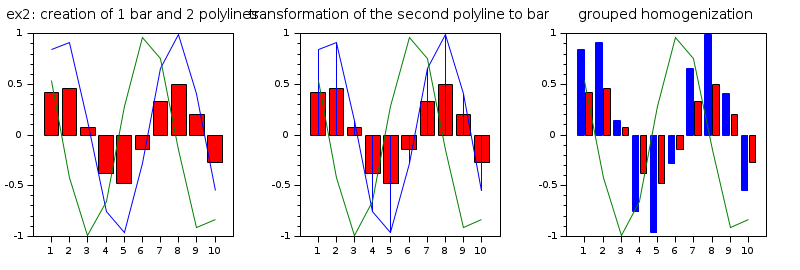Scilab Home page | Wiki | Bug tracker | Forge | Mailing list archives | ATOMS | File exchange
scilab-6.1.1
Change language to: English - Français - Português - 日本語 -
Справка Scilab >> Графики > bar_histogram > barhomogenize

# barhomogenize

homogenize all the bars included in the current working axes

### Syntax

```barhomogenize()
barhomogenize(h)
barhomogenize([h,] style)
barhomogenize([h,] width)
barhomogenize([h,] style, width)```

### Arguments

h

an axes handle: The axes where barhomogenize() must be applied. Default `h=gca()`.

style

word `'grouped'` (default) or `'stacked'`

width

a decimal number `0 < width <= 1`, setting the width of bars, as a fraction of the maximum allowed width. Default: 0.8.

### Description

If there are several bar calls, the `barhomogenize` function allows to homogenize the width and style of all bars (i.e which has the `polyline_style` type 6) included in the current working axes. These bars must have the same x data.

`barhomogenize( ) :` takes the default values ```h=gca(), width=0.8, style='grouped'.```

`barhomogenize(h,...) :` defines the current axes where the drawing is performed.

`barhomogenize(...,style,...) :` defines how the bars are drawn. The 'grouped' option allows to center the M polylines versus each components of x, and the 'stacked' option allows to stack them.

`barhomogenize(...,width) :` defines the width of the bar(s) in percentage (generally: 0<width<=1).

### Examples

First example: creation of 1 yellow bar (i.e 1 polyline with polyline_style=6) and 3 bars (i.e 3 polylines with polyline_style=6)

```subplot(2,3,1)
xtitle('ex1: creation of 1 yellow bar and 3 bars')
x=1:3;
y1=1:3;
y2=[4 3 5;6 7 8;9 10 11];
bar(x,y1,'yellow');bar(x,y2);

// grouped homogenization of these 4 bars
subplot(2,3,2)
xtitle('grouped homogenization')
x=1:3;
y1=1:3;
y2=[4 3 5;6 7 8;9 10 11];
bar(x,y1,'yellow');bar(x,y2);
barhomogenize();

// stacked homogenization of the 4 bars
subplot(2,3,3)
xtitle('stacked homogenization')
x=1:3; y1=1:3; y2=[4 3 5;6 7 8;9 10 11];
bar(x,y1,'yellow');bar(x,y2);
barhomogenize('stacked',1);```Second example :creation of 1 red bar (i.e 1 polyline with polyline_style=6) and 2 polylines with type=1 (calling plot function).

```subplot(2,3,4)
xtitle('ex2: creation of 1 bar and 2 polylines')
x=1:10;
y=sin(x)/2;
bar(x,y,'red')
x1=1:10;
y1=[sin(x);cos(x)]
plot(x1',y1')

// modify the polyline_style type of the second polyline from plot (this polyline becomes a bar)
subplot(2,3,5)
xtitle('transformation of the second polyline to bar')
x=1:10;
y=sin(x)/2;
bar(x,y,'red')
x1=1:10;
y1=[sin(x);cos(x)]
plot(x1',y1')
e=gce();
e2=e.children(2);
e2.polyline_style=6;

// homogenization of the first bar (from bar function) and second bar (from the modification).
subplot(2,3,6)
xtitle('grouped homogenization')
x=1:10;
y=sin(x)/2;
bar(x,y,'red')
x1=1:10;
y1=[sin(x);cos(x)]
plot(x1',y1')
e=gce();
e2=e.children(2);
e2.polyline_style=6;
barhomogenize();
// change the style and the width
//barhomogenize('stacked',0.5);
//barhomogenize('stacked',1);```